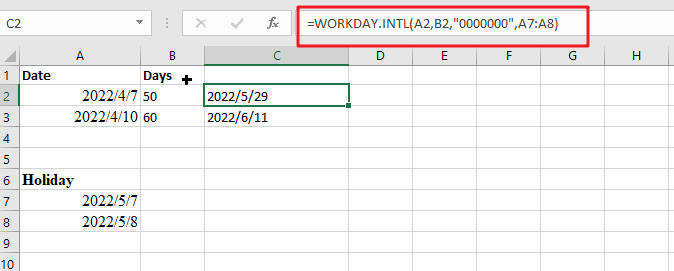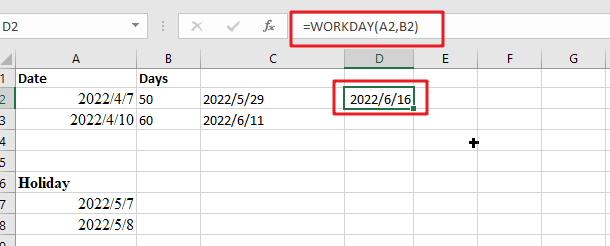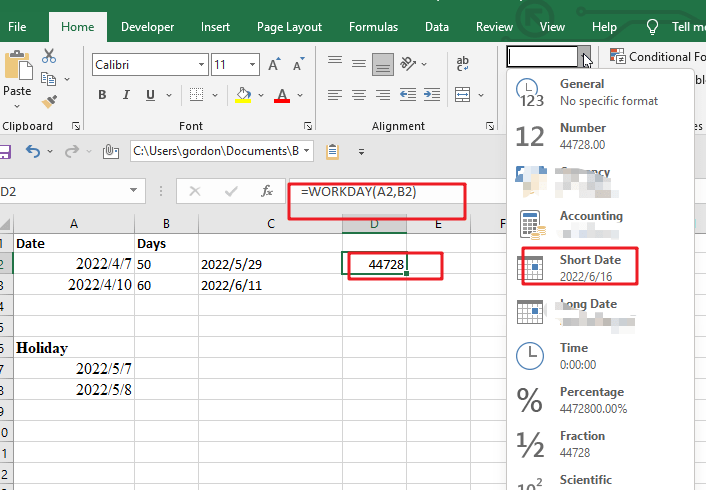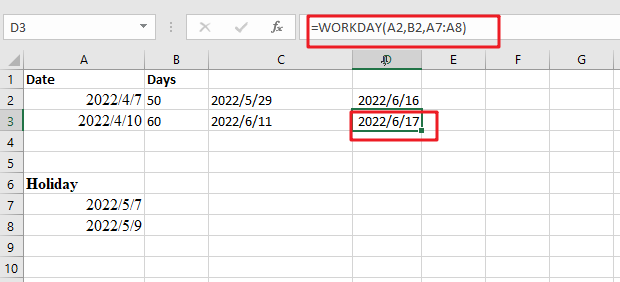# Add Workdays in Excel

To add or subtract workdays days from a date, respecting holidays but assuming a 7-day week: you can use the WORKDAY.INTL function with an argument that tells it how many days should be subtracted/added as well as which specific holidays to ignore. In our formula below C2 for this example:

`=WORKDAY.INTL(A2,B2,"0000000",A7:A8)`With this formula, you can have a Thursday evening deadline instead of Monday afternoon! This means that all days in the week are treated as working hours. With two holidays  in range A7:A8  and weekends set using the special syntax “0000000“, your work will be done by 5/29/2022, at midnight PST.

## Explanation

WORKDAY.INTL allows you to calculate a date in the future or past that respected holidays and weekends with its special codes for designating which days are considered as such, but using masks is more flexible since it allows any day of the week to be designated into being treated like weekend ones & zeros!

You can calculate the number of days required to complete your project in an official workplace with this handy formula. Simply multiply Saturday’s total hours by 0,8 and add it onto Sunday-Friday count!

To add days excluding weekends, simply do the following:

Type this formula =WORKDAY(A2, B2) into the blank cell, and press Enter key to get your answer.Tip: To change the start date of your spreadsheet, simply enter “A2” as desired. This will allow you to input future dates into B2 for each day added on top!

We have now reached the end date, which adds 50 business days, excluding weekends.

Note: The number you’re trying to math is a 5-digit one, so in order for it to show up on your screen correctly formatted as date, click Home > Number Format > Short Date. See screenshot:Now that you have cells containing all of your holiday data, excluding weekends and any other days we want is easy.

Type this formula =WORKDAY(A2, B2, A7:A8) in blank cells and hit the enter to see the result.Tip: To create a formula that excludes holidays, use this equation. A2 is the start date, and A7:A8 are days you want to be excluded from your calculation.

### Related Functions

• Excel WORKDAY function
The Excel WORKDAY function returns a serial number that represents a date that is the indicated number of working days before or after the starting date you specified.The syntax of the WORKDAY function is as below:=WORKDAY(start_date, days, [holidays])…
• Excel WORKDAY.INTL function
The Excel WORKDAY.INTL function returns the serial number of the date before or after a specified number of workdays.The syntax of the WORKDAY.INTL function is as below:= WORKDAY.INTL (start_date, days, [weekend], [holidays])…
Related Posts

Sort/Rank Numeric Values with Duplicate Values Exist

Excel built-in RANK function can sort a set of values. If there are duplicate numbers, then the rank number is also duplicated. See the following example: There are two numbers “100” in range A2:A9, they are both the third largest ...

Calculate Days Open in Excel

If you want to know how to Calculate days in Excel, there are some formulas that you can use to do so. For example, you can use the DAYS function in Excel to find the number of days between two ...

Calculate Grades With VLookup in Excel

Why Should You Calculate Grades With VLookup Excel? If you're looking for a simple way to find out a student's grades, VLOOKUP Excel can do the trick. This function uses a lookup table to find the values and sort them ...

Repeating Character n Times in Excel

In daily life, we can use repeated characters to indicate the magnitude or priority of something. For example, a single “!” indicates a minor case, double “!!” indicates a medium case, and triple “!!!” indicates a high case, and so ...

Calculate Cumulative Loan Interest in Excel

What is Cumulative Loan Interest? When comparing different types of loans, many people want to know what is Cumulative Loan Interest. The sum of all interest payments you've made on a loan is referred to as cumulative interest. Different lenders ...

Sidebar